﻿ 双端固支谐振梁动态响应分析

# 双端固支谐振梁动态响应分析Dynamic Response Analysis of Double-Ended Resonant Beams

Abstract: The resonant sensor has attracted more attention because of its high sensitivity, small footprint, stability, compatibility with multiple-phase samples. The typical resonant sensor measures reso-nant frequency modulated by axial load to realize mass sensing. Dynamic characteristic is an im-portant factor to evaluate stability of resonant beam, and the key point is to analyze the dynamic response of the resonant beam under dynamic axial load. The article is based on infinitesimal method; mathematical model of vibration of resonant beam impacted by dynamic axial force was established through the resonant beam micro mechanical balance and analyzed through Mathieu equation. Equivalent circuit method was chosen to solve vibration model. The simulation of the circuit shows the dynamic response of resonant beam under the typical axial load. Besides, the article studied the effect of damping on the resonant beam and concluded the damping only influenced on vibration amplitude scarcely on frequency.

1. 引言

2. 谐振梁振动数学模型建立

F——梁承受的载荷；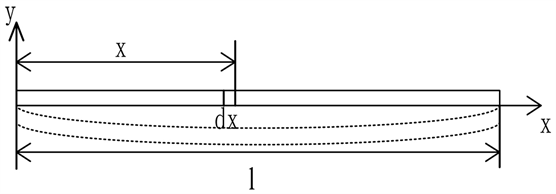Figure 1. Schematic diagram of bending vibration of double end fixed beam under dynamic axial force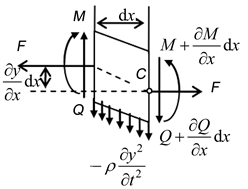Figure 2. Force analysis of the micro sec- tion dx of the resonant beam

$\rho$ ——梁的密度；

E——梁的弹性模量；

I——梁横截面对中性轴的惯性矩。

A、L、h、b分别是梁的横截面积、长度、高度、宽度。设 $y\left(x,t\right)$ 表示谐振梁上距原点为x处的截面在t时刻的横向位移，由力矩平衡公式与弯矩平衡公式共同联立得梁横向弯曲振动的方程为：

$EI\frac{{\partial }^{4}y}{\partial {x}^{4}}-F\frac{{\partial }^{2}y}{\partial {x}^{2}}+\rho A\frac{{\partial }^{2}y}{\partial {t}^{2}}=0$ (1)

$y\left(x,t\right)=\varphi \left(x\right)\mathrm{sin}\left(\omega t+\phi \right)$ (2)

$EI\frac{{\text{d}}^{4}Y}{\text{d}{x}^{4}}-F\frac{{\text{d}}^{2}Y}{\text{d}{x}^{2}}-\rho AY\frac{{\text{d}}^{2}P}{\text{d}{t}^{2}}=0$ (3)

${\phi }_{i}\left(x\right)=\text{ch}{\lambda }_{i}x-\mathrm{cos}{\lambda }_{i}x+{\eta }_{i}\left(\text{sh}{\lambda }_{i}x-\mathrm{sin}{\lambda }_{i}x\right)$ (4)

$\left\{\begin{array}{l}\frac{{\text{d}}^{2}Y\left(0\right)}{\text{d}{x}^{2}}=0\\ \frac{{\text{d}}^{2}Y\left(l\right)}{\text{d}{x}^{2}}=0\\ EI\left[\frac{{\text{d}}^{3}Y\left(0\right)}{\text{d}{x}^{3}}\right]=kY\left(0\right)\\ EI\left[\frac{{\text{d}}^{3}Y\left(l\right)}{\text{d}{x}^{3}}\right]=kY\left(l\right)\end{array}$ (5)

$\mathrm{cos}\lambda l\text{ }\text{ }\text{ch}\lambda l+1=-\frac{k}{EI{\lambda }^{3}}\left(\mathrm{cos}\lambda l\text{ }\text{ }\text{sh}\lambda l-\mathrm{sin}\lambda l\text{ }\text{ }\text{ch}\lambda l\right)$ (6)

${\lambda }_{1}l=1.880$ (7)

${\eta }_{1}=\frac{\mathrm{cos}\lambda L-\text{ch}\lambda L}{\text{sh}\lambda L-\mathrm{sin}\lambda L}$ (8)

$\varphi \left(x\right)=\mathrm{ch}\lambda x+\mathrm{cos}\lambda x+0.7341\left(\mathrm{sh}\lambda x+\mathrm{sin}\lambda x\right)$ (9)

$\left(\underset{0}{\overset{l}{\int }}\rho A{\varphi }^{2}\text{d}x\right)\frac{{\text{d}}^{2}P}{\text{d}{t}^{2}}+\left(\underset{0}{\overset{l}{\int }}EI{\left({\varphi }^{″}\right)}^{2}\text{d}x-F\left(t\right)\underset{0}{\overset{l}{\int }}{\left({\varphi }^{\prime }\right)}^{2}\text{d}x\right)\cdot P=0$ (10)

$\frac{{\text{d}}^{2}P}{\text{d}{t}^{2}}+\left(A-B×F\left(t\right)\right)\cdot P=0$ (11)

$\left\{\begin{array}{l}\stackrel{˜}{K}=\frac{\underset{0}{\overset{l}{\int }}EJ{\left({\varphi }^{″}\right)}^{2}\text{d}x}{\underset{0}{\overset{l}{\int }}\rho A{\varphi }^{2}\text{d}x}\\ \stackrel{˜}{\alpha }=\frac{\epsilon \cdot \underset{0}{\overset{l}{\int }}{\left({\varphi }^{\prime }\right)}^{2}\text{d}x}{2\underset{0}{\overset{l}{\int }}\rho A{\varphi }^{2}\text{d}x}\end{array}$ (12)

$\frac{{\text{d}}^{2}P}{\text{d}{t}^{2}}+\left(\stackrel{˜}{K}-2\stackrel{˜}{\alpha }\mathrm{cos}\left(\omega \cdot t\right)\right)P=0$ (13)

$\frac{{\text{d}}^{2}P}{\text{d}{t}^{2}}+\left(3.0434×{10}^{11}-N\left(t\right)×5.2798×{10}^{12}\right)\cdot P=0$ (14)

3. 谐振梁振动模型电路模拟

4. 阻尼对谐振梁振动特性影响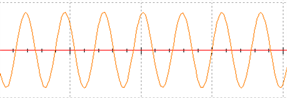(a) 载荷为0 mV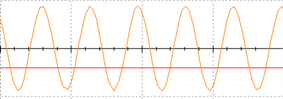(b) 载荷为−20 mV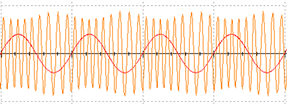(c) 载荷频率为10 kHz幅值为10 mv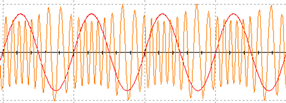(d) 载荷频率为10 kHz幅值为20 mV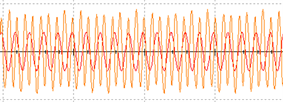(e) 载荷频率为50 kHz幅度为10 mv

Figure 4. Circuit simulation results

① 等效电路方法分析

$\frac{{\mathrm{d}}^{2}P}{\mathrm{d}{t}^{2}}+c\cdot \frac{\mathrm{d}P}{\mathrm{d}t}+\left(A-B×F\left(t\right)\right)\cdot P=0$ (15)

$\left\{\begin{array}{l}\frac{-1}{{C}_{1}{R}_{1}}\underset{0}{\overset{t}{\int }}-\left(\left(A-B×F\left(t\right)\right)\cdot P+{k}^{\prime }{u}_{1}\left(t\right)\right)\text{d}t={u}_{1}\left(t\right)\\ \frac{-1}{{C}_{2}{R}_{2}}\underset{0}{\overset{t}{\int }}{u}_{1}\left(t\right)\text{d}t=P\end{array}$ (16)

$\frac{{\text{d}}^{2}P}{\text{d}{t}^{2}}+\frac{{k}^{\prime }}{{R}_{1}{C}_{1}}\cdot \frac{\text{d}P}{\text{d}t}+\frac{1}{{R}_{1}{C}_{1}{R}_{2}{C}_{2}}\left(A-B×F\left(t\right)\right)\cdot P=0$ (17)

② COMSOL方法分析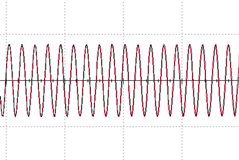(a) 载荷为0 mV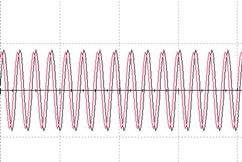(b) 载荷为−20 mV(c) 载荷频率为10 kHz幅值为10 mv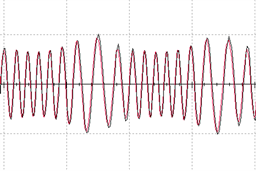(d) 载荷频率为10 kHz幅值为20 mV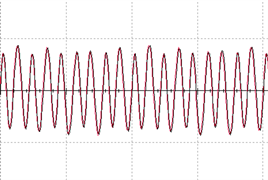(e) 载荷频率为50 kHz幅度为10 mv

Figure 6. Circuit with damping simulation results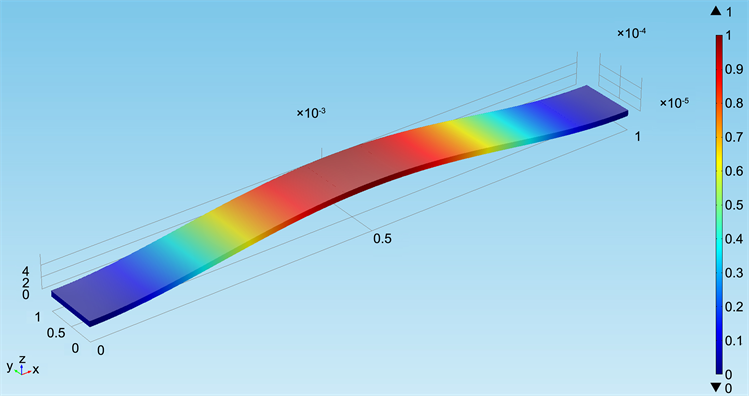Figure 7. Simulation of resonant beam’s amplitude by comsol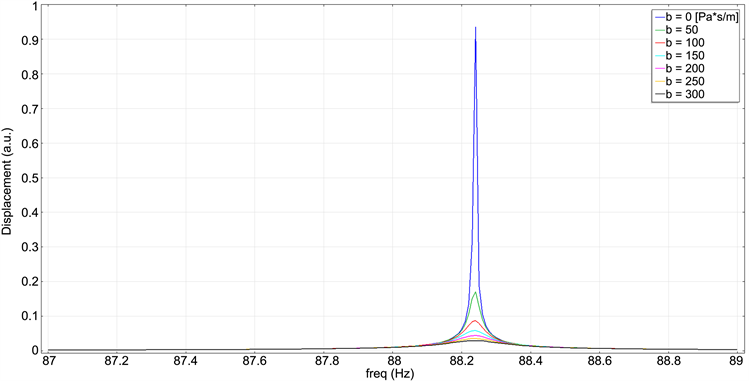Figure 8. Simulation of resonant beam’s frequency by comsol

5. 结论

 樊尚春. 传感器技术及应用[M]. 北京: 北京航空航天大学出版社, 2004.

 邢维巍, 张硕, 樊尚春. 动态轴向载荷下谐振梁振动响应分析[J]. 传感技术学报, 2016, 29(9): 1372-1375.

 Piccardo, G. and Tubino, F. (2012) Dynamic Response of Euler-Bernoulli Beams to Resonant Harmonic Moving Loads. Structural Engineering and Mechanics, 5, 681-704.

 Piccardo, G., Ranzi, G. and Luongo, A. (2014) A Complete Dynamic Approach to the Generalized Beam Theory Cross-Section Analysis including Extension and Shear Modes. Mathematics and Mechanics of Solids, 19, 900-924.
https://doi.org/10.1177/1081286513493107

 Mclachlan, N.W. (1964) Theory and Application of Mathieu Functions. Dover Publicatons, Inc.

 Silvestre, N. and Camotim, D. (2013) Shear Deformable Generalized Beam Theory for the Analysis of Thin-Walled Composite Members. Journal of Engineering Mechanics, 139, 1010-1024.

 周建发, 杨贵玉. 一种MEMS谐振梁的热弹阻尼分析[J]. 仪表技术与传感器, 2009(b11): 84-86.

 凌源. RF MEMS自由梁谐振器特性研究[D]: [硕士学位论文]. 成都: 电子科技大学, 2014.

 续龙, 樊尚春, 曹乐, 等. 基于模拟电路方法的Mathieu方程特性研究[C]//中国仪器仪表学会. 全国敏感元件与传感器学术会议: 2014年卷. 北京: 国防工业出版社, 2014.

Top